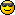<![CDATA[Math Is Fun Forum / Logarithms]]> 2017-09-24T23:06:31Z FluxBB http://www.mathisfunforum.com/viewtopic.php?id=3113 <![CDATA[Re: Logarithms]]> JaneFairfax wrote:

JaneFairfax, here is a basic proof of L4:

For all real a > 1, y = a^x is a strictly increasing function.

log(base 2)3 versus log(base 3)5

2*log(base 2)3 versus 2*log(base 3)5

log(base 2)9 versus log(base 3)25

2^3 = 8 < 9

2^(> 3) = 9

3^3 = 27 > 25

3^(< 3) = 25

So, the left-hand side is greater than the right-hand side, because
Its logarithm is a larger number.

]]>
http://www.mathisfunforum.com/profile.php?id=213376 2017-09-24T23:06:31Z http://www.mathisfunforum.com/viewtopic.php?pid=400816#p400816
<![CDATA[Re: Logarithms]]> Hi all,

I saw this post today and saw the probs on log. Well, they are not bad, they are good. But you can also try these problems here by me (Credit: to a book):
http://www.mathisfunforum.com/viewtopic … 93#p399193

]]>
http://www.mathisfunforum.com/profile.php?id=213230 2017-07-02T14:41:41Z http://www.mathisfunforum.com/viewtopic.php?pid=400013#p400013

This problem was posted by JaneFairfax. I think it would be appropriate she verify the solution.

]]>
http://www.mathisfunforum.com/profile.php?id=682 2011-05-28T11:57:00Z http://www.mathisfunforum.com/viewtopic.php?pid=175488#p175488
<![CDATA[Re: Logarithms]]> JaneFairfax wrote:

L # 4

I don't want a method that will rely on defining certain functions, taking derivatives,
noting concavity, etc.

Change of base:

Each side is positive, and multiplying by the positive denominator
keeps whatever direction of the alleged inequality the same direction:

On the right-hand side, the first factor is equal to a positive number less than 1,
while the second factor is equal to a positive number greater than 1.  These
facts are by inspection combined with the nature of exponents/logarithms.

Because of (log A)B = B(log A) = log(A^B), I may turn this into:

I need to show that

Then

Then 1 (on the left-hand side) will be greater than the value on the
right-hand side, and the truth of the original inequality will be established.

I want to show

Raise a base of 3 to each side:

Each side is positive, and I can square each side:

-----------------------------------------------------------------------------------

Then I want to show that when 2 is raised to a number equal to
(or less than) 1.5, then it is less than 3.

Each side is positive, and I can square each side:

]]>
http://www.mathisfunforum.com/profile.php?id=116930 2011-05-28T07:59:31Z http://www.mathisfunforum.com/viewtopic.php?pid=175475#p175475
<![CDATA[Re: Logarithms]]> log_2(16) = \log_2 \left ( \frac{64}{4} \right ) = \log_2(64) - \log_2(4) = 6 - 2 = 4, \,

log_2(\sqrt4) = \frac {1}{3} \log_2 (4) = \frac {2}{3}. \,

]]>
http://www.mathisfunforum.com/profile.php?id=72549 2010-08-17T04:15:11Z http://www.mathisfunforum.com/viewtopic.php?pid=148322#p148322
<![CDATA[Re: Logarithms]]> Gentleman,

Thanks for the proofs.
Regards.

]]>
http://www.mathisfunforum.com/profile.php?id=682 2010-04-19T13:02:18Z http://www.mathisfunforum.com/viewtopic.php?pid=134625#p134625
<![CDATA[Re: Logarithms]]> Hi ganesh
for  L # 2
I think that  the following proof  is easier:
Assume Log(x)/(b-c)=Log(y)/(c-a)=Log(z)/(a-b)=t
So Log(x)=t(b-c),Log(y)=t(c-a)  ,  Log(z)=t(a-b) So Log(x)+Log(y)+Log(z)=tb-tc+tc-ta+ta-tb=0
So Log(xyz)=0 so   xyz=1   Q.E.D
Best Regards

]]>
http://www.mathisfunforum.com/profile.php?id=43149 2010-04-19T09:14:13Z http://www.mathisfunforum.com/viewtopic.php?pid=134611#p134611
<![CDATA[Re: Logarithms]]> Hi ganesh
for  L # 1
since log(a)= 1 / log(b),    log(a)=1
b               a            a
we have
1/log(abc)+1/log(abc)+1/log(abc)=
a                 b                c
log(a)+log(b)+log(c)= log(abc)=1
abc      abc          abc    abc
Best Regards

]]>
http://www.mathisfunforum.com/profile.php?id=43149 2010-04-19T09:04:41Z http://www.mathisfunforum.com/viewtopic.php?pid=134610#p134610
<![CDATA[Re: Logarithms]]> log a = 2log x + 3log y

b = log x  log y

log a + 3 b = 5log x

loga - 2b = 3logy + 2logy = 5logy

logx / logy = (loga+3b) / (loga-2b)

]]>
http://www.mathisfunforum.com/profile.php?id=62067 2010-04-19T07:06:39Z http://www.mathisfunforum.com/viewtopic.php?pid=134586#p134586
<![CDATA[Re: Logarithms]]>

]]>
http://www.mathisfunforum.com/profile.php?id=6777 2009-02-07T11:31:40Z http://www.mathisfunforum.com/viewtopic.php?pid=104602#p104602
<![CDATA[Re: Logarithms]]> You still used a calculator / log table in the past to get those figures (or someone else did and showed them to you). I say again: no calculators or log tables to be used (directly or indirectly) at all!!]]>
http://www.mathisfunforum.com/profile.php?id=6777 2009-01-06T09:57:49Z http://www.mathisfunforum.com/viewtopic.php?pid=101437#p101437
<![CDATA[Re: Logarithms]]> No, I didn'tI remember

and
.

]]>
http://www.mathisfunforum.com/profile.php?id=682 2009-01-05T13:36:33Z http://www.mathisfunforum.com/viewtopic.php?pid=101407#p101407
<![CDATA[Re: Logarithms]]> You are not supposed to use a calculator or log tables for L # 4.Try again!

]]>
http://www.mathisfunforum.com/profile.php?id=6777 2009-01-05T11:37:30Z http://www.mathisfunforum.com/viewtopic.php?pid=101391#p101391
<![CDATA[Re: Logarithms]]>

]]>
http://www.mathisfunforum.com/profile.php?id=682 2009-01-05T04:13:57Z http://www.mathisfunforum.com/viewtopic.php?pid=101378#p101378
<![CDATA[Re: Logarithms]]> L # 4

]]>
http://www.mathisfunforum.com/profile.php?id=6777 2009-01-04T23:58:47Z http://www.mathisfunforum.com/viewtopic.php?pid=101358#p101358